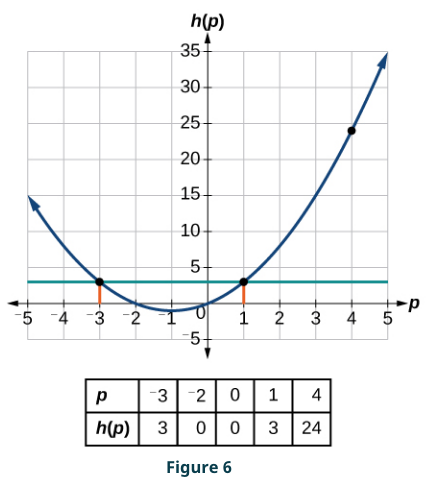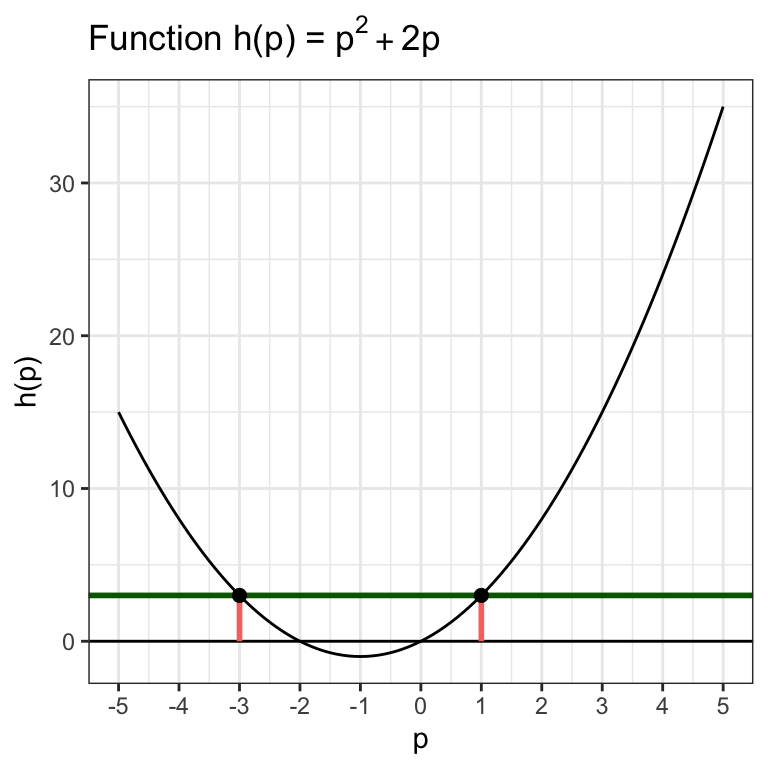# Precalculus: 01 Function

Author

Peter Baumgartner

Published

2023-10-19 23:31

# Preface

This is work in progress: Finished until p.34 of 162 pages for chapter “Function”.

This Quarto book collects my personal notes, trials and exercises for the first chapter “Function” of Abramson, J. (2021). Precalculus 2e (2nd ed.). OpenStax College.

Because of the huge amount of the material in the book I am planning a separate Quarto book for each of the 12 chapters of Precalcuclus. (So the plan: I am not sure if I will ever finish this monster project 😜)

## Structure of the Quarto book

### R Code

Sometimes I use R Code for calculation or visualization tasks.

R is a free software environment for statistical computing and graphics. It compiles and runs on a wide variety of UNIX platforms, Windows and MacOS. To download R, please choose your preferred CRAN mirror. (The R Project for Statistical Computing)

R Code 1 : For a better understanding I replicated Figure 6, p.16.Graph 1: $$h(p) = p^2 + 2p$$ – This is the original [Figure 6, p.16]

Listing 1: Plot function $$h(p) = p^2 + 2p$$

ggplot2::ggplot() +
ggplot2::scale_x_continuous(limits = c(-5, 5), breaks = seq(-5, 5, 1)) +
ggplot2::geom_function(fun = function(x) x^2 + (2 * x)) +
ggplot2::geom_hline(yintercept = 0, linetype = "solid") +
ggplot2::geom_hline(yintercept = 3, linetype = "solid",
color = "darkgreen", linewidth = 1) +
ggplot2::geom_segment(ggplot2::aes(x = -3, y = 0, xend = -3, yend = +3,
color = "orange"), show.legend = FALSE, linewidth = 1) +
ggplot2::geom_segment(ggplot2::aes(x = 1, y = 0, xend = 1, yend = +3,
color = "orange"), show.legend = FALSE, linewidth = 1) +
ggplot2::geom_point(ggplot2::aes(x = -3, y = +3,
fill = "blue"), size = 2, show.legend = FALSE) +
ggplot2::geom_point(ggplot2::aes(x = 1, y = +3,
fill = "blue"), size = 2, show.legend = FALSE) +
ggplot2::theme_bw() +
ggplot2::labs(
title = expression(paste("Function h(p) = ", p^2 + 2 * p)),
x = "p",
y = "h(p)"
)Graph 2: Graph for the function $$h(p) = p^2 + 2p$$ showing the solution for $$h(p) = 3$$

Compare my graph by hovering with the mouse over the link to the original Graph 1.

### Exercises

Solving the exercises I use the following procedure:

Procedure 1 : Solving Exercises

1. As my main goal is learning I am trying to solve only the odd numbered exercises where an official solution is available.
2. At first I write my own solution in the appropriate exercise callout and compare it with the official solution.
3. If it is correct: Fine! If not, I am trying to understand why I failed. If I am stuck and I can’t understand the solution I am looking for other resources.
4. If I succeed I will substitute in the exercise callout my wrong solution with the correct one.
5. Additionally I will add a separate “Watch Out!” callout where I present my wrong attempt with an explication why I failed. I am not sure if this part is helpful for other people but to express explicitly what went wrong turned out of immense value for my personal learning.

### Used Resources

To support my learning and especially to consult if I could not find a solution I used the following resources:

Additional resources I have used for this Quarto book

• Khan Academy: Trying to solve the exercises I noticed big knowledge gaps in my Math education, especially with factoring and transforming of quadratic equations and functions. I interrupted my reading and practicing of the Precalculus book and worked through Unit 13: Quadratics: Multiplying & factoring and the first two lessons of Unit 14: Quadratic functions & equations from the Algebra 1 course. If I will notice during my reading of Precalculus that I need to visit other lessons / units as well, I will report it here.
• math.stackexchange: Sometimes people asked exactly the exercise questions on math.stackexchange, where I could find helpful answers.
• MathePower: There are free online math calculator on the internet. MathePower is one of it. It has the nice feature that you not get the solutions but also explications for every step of the calculation.
• WolframAlpha: WolframAlpha is a specialized online service that not only works like a semantic search engine but uses Wolfram Language a proprietary programming language for symbolic computation and functional programming. In the free version it does not have step-by-step explications but is plotting the graph and displays not only the solution but also alternative forms of the equation formula.
• The Glaser Tutoring Company: I counted 643 videos only for Math(!) and – as far as I have seen – the majority of them uses the exercises of the Precalculus book. The videos are pedagogically well done and explain every detail of the exercise.